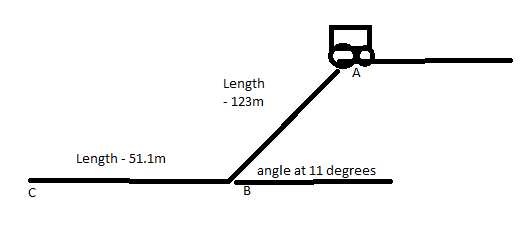Cart Problem

Homework Statement

Two guys are sitting in a cart. At point C, they jump off to avoid the cliff below.
How long in time(total) will it take the guys to go from Point A to C?
How long will it take them to roll from A to B, and from B to C?Homework Equations

t = square root[2*(l)/(g)(sinө)] <-- given equation for measuring time taken for object to go down an inclined plane

The Attempt at a Solution

Given the equation, I found that time taken from A to B is about 11.46s, using the formula and the given values: 123m, g = 9.81m/s^2, and 11 degrees. For the part where there is a flat surface, I am confused as to how to determine time. I found out velocity from A to B to be 10.69m/s using d/t. Would I be able to use this velocity and the second distance given to find time between B to C?

Is the velocity of the cart constant from point B to C, seeing that it doesn't stop when reaching the edge of the cliff?

gneill
Mentor
The cart is accelerating from A to B, so what you've determined is an average velocity for that leg of the journey. It won't be the velocity that the cart has when it reaches B.

To find the velocity at B you have a couple of options. Either employ conservation of energy to figure how the drop in height converts the potential energy to kinetic energy, or employ your acceleration and time in a kinematic equation to determine the velocity.

Once you have the velocity at B, assume it remains constant across the level path from B to C and work out the time to traverse it.

So you are saying Et = Et'
Meaning the energy before = energy after
In this case there was potential energy before w/ no kinetic, and then kinetic energy after with no potential energy.

m(g)(h) = 1/2mv^2
cancel out m and solve for v?

gneill
Mentor
Sure. Why not try it both ways and see if you get the same result?

Wait I don't understand the other way you said we can approach the problem.
"employ your acceleration and time in a kinematic equation to determine the velocity." <-- how would you do this?

gneill
Mentor
Wait I don't understand the other way you said we can approach the problem.
"employ your acceleration and time in a kinematic equation to determine the velocity." <-- how would you do this?

What's the downslope acceleration of the cart? You used it to find the time to go from A to B in your first post. d = (1/2)at2